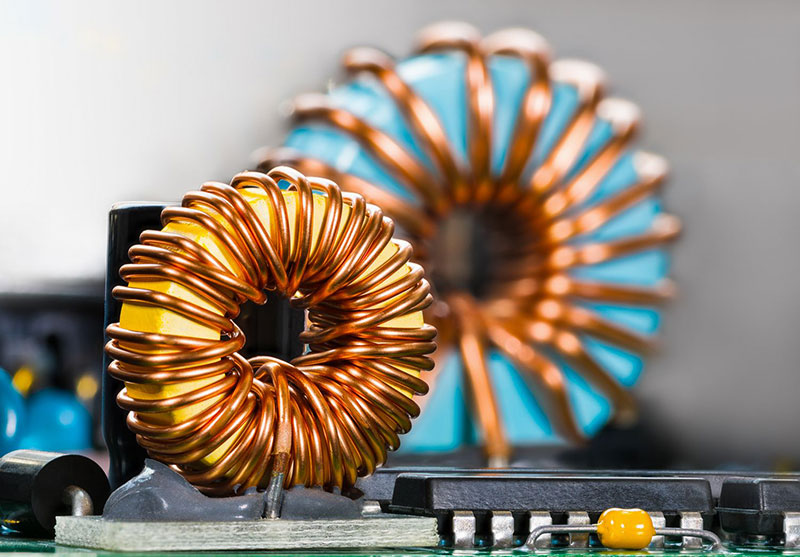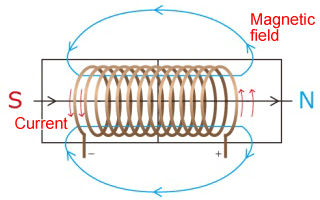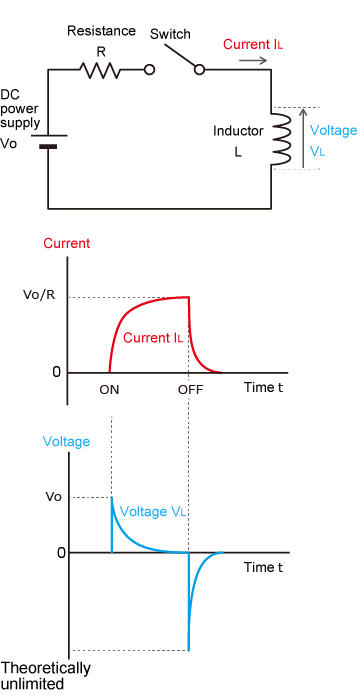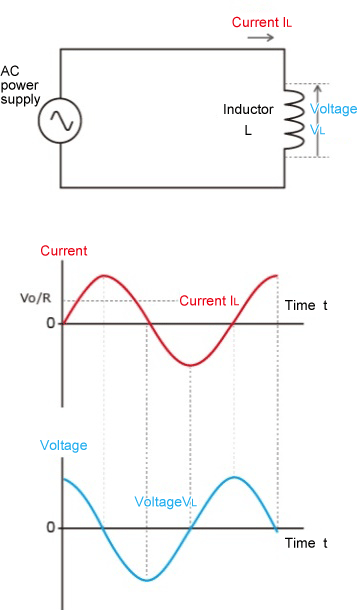# Tech Tips## What is an inductor (coil)?

Inductors are called passive components, the same as resistors (R) and capacitors (C), and are electronic components labeled with an "L". It has the function of keeping the current constant. The ability of an inductor is expressed by "inductance". The unit is henry (H).

An inductor has the same structure as a coil, but most inductors called inductors have a single winding (1 roll). Some are wound only with conductors, while others have a core inside the wound conductors. The action of an inductor is proportional to the square of the number of turns or radius, and inversely proportional to the length.

### Basic principles of inductors

First of all, let's briefly explain the principle of inductors. When an electric current flows through a conductor, a magnetic force is generated around it in the direction of the right-hand thread. When current flows through an inductor with conductors wrapped around it in the same direction, the magnetic field generated around the wire is bound together and becomes an electromagnet (Figure 1). Conversely, it is also possible to generate an electric current from magnetic force.Figure (1)Figure (2)Figure (3)

Principle of inductors

When a magnet is moved closer or further away from an inductor that has become an electromagnet, the magnetic field of the inductor changes. This causes an electric current to flow in order to generate a "force against change" that tries to maintain the direction and momentum of the magnetic field. This is called "electromagnetic induction.

As shown in the circuit diagram, when a DC current flows through an inductor (Figure 2), an electromotive force in the direction that interferes with the current is generated at the beginning of the current flow. This property is called the self-inductive effect. However, later on, as the DC current reaches a certain value, the magnetic flux ceases to change and the electromotive force is no longer generated, thus the current is no longer obstructed.

The electromotive force generated in an inductor is proportional to the rate of change of the current (ΔI /Δt) .

V＝L・ΔI／Δt

V: Electromotive force (V)
L: Inductance (H)
ΔI /Δt: Rate of change of current (A/s)

On the other hand, when AC current is applied (Fig. 3), the voltage becomes larger when the current rises from 0 because the rate of change of current is the largest. As the rate of current increase slows down, the voltage decreases, and at the point where the current reaches its maximum, the voltage becomes zero.

As the current begins to fall from its maximum value, a negative voltage begins to be generated, and the voltage is at its lowest point when the current reaches zero. Looking at the waveforms of the voltage and current here, we can see that the electromotive force is generated with a phase that is 1/4 slower.

Therefore, AC current is more difficult to pass than DC current. Furthermore, if the frequency of AC exceeds a certain value, the current will be constantly blocked by the electromotive force, and the current will not flow. Therefore, the higher the frequency of the AC voltage, the more difficult it is for the current to flow.

To summarize

• When current flows, magnetic force is generated.
• When the magnetic field changes, current flows
• It is easy to pass direct current and difficult to pass alternating current.

Because of these properties, inductors are used in a variety of applications.

## Roles of inductors (coils)

### 1. Applications for power circuits

As mentioned above, inductors can easily pass DC current, but they have a property that makes it difficult to pass AC current. In addition, when passing AC current, inductors have the property of suppressing its waves and transforming it into a smoother current. For this reason, inductors are used in power supply circuits for electronic circuits that run on DC current.

Ordinary power supplies are AC circuits, so in order to operate electronic circuits, it is necessary to pass through a smoothing circuit to adjust the current. Inductors are used in these smoothing circuits. Inductors are also useful in removing noise due to their ability to keep out high frequency AC. Inductors used in power supply circuits are mainly called power inductors or choke coils.

### 2. Applications for high-frequency circuits

The basic mechanism and concept of inductors for high frequency circuits are the same as those for power supply circuits. However, the high-frequency circuits that are often used for communications such as wireless LAN are in the high-frequency band from several tens of MHz to several GHz, so ordinary inductors cannot be used in such circuits. Therefore, inductors with higher performance (Q-value: quality factor) than ordinary inductors are used.

Ideally, an inductor should have only the function of inductance, but in reality, it has internal and terminal resistance, and it also has distribution capacitance and other characteristics that cause the coils to act like the electrodes of a capacitor.

Capacitors are the opposite of inductors in that they have the property of passing AC current without passing DC current. Therefore, when the frequency is low, the characteristics of an inductor prevail, but when the frequency exceeds a certain level, the function as a capacitor prevails over the function as an inductor, and it can no longer be used as an inductor.

The frequency at which this reversal occurs is called the self-resonant frequency. When a current with a frequency close to the self-resonant frequency flows, the properties of the inductor and the properties of the capacitor cancel each other out. As a result, the impedance (resistance in AC circuits) of the inductor decreases, and more current can flow. Taking advantage of this property, inductors for high-frequency circuits are used for the purpose of extracting signals with specific frequencies.

### 3. Applications for power transformer

Inductors are also used in transformers installed on utility poles and the like. In transformer applications, they are not called inductors but coils more often. When an AC voltage is applied to an inductor, the current flowing through it changes, which causes the magnetic force to change, and this magnetic force affects the surrounding inductors, generating a voltage. This kind of action is called "mutual induction.

In a transformer, the change in magnetic force created by the current flowing through a coil with a large number of turns is affected by a nearby coil with a smaller number of turns, thereby generating a larger voltage and raising the voltage.

In addition to converting voltage for power circuits, there are other types of inductors used in radio and wireless circuits, such as "IFTs" that extract intermediate frequency signals, and "audio transformers" that convert audio frequency signals.

## Types of inductors (coils)

Next, let's look at the main inductor classifications and their characteristics. There are many ways to classify them, but here we will first classify them according to the structure of the winding.

### 1. Wire-wound inductor

A wire-wound inductor is an inductor that is closest in shape to a coil, with the conductor wound in a spiral shape as explained in the first section. Some inductors are hollow, while others have conductors wrapped around a core (like a bobbin used in sewing machines). There are a variety of sizes and shapes depending on the application and inductance value.

They are suitable for circuits where a large current must flow or where a high inductance value is required.

### 2.Multilayer inductors

Multilayer inductors are made of alternating layers of ferrite or ceramic and coil patterns. The coil pattern is not made by winding conductors, but by screen-printing conductors on top of the ferrite or other material. Layers and layers of this are used to give it the properties of a coil. On the other hand, due to its structure, it also has a capacitor component inside.

### Inductors have different names depending on their use

Inductors are used in a variety of places in our daily lives. Depending on the application, they are called coils, choke, reactor, solenoids, line filters, etc., as in the case of transformers. The following is a list of typical names.

#### Choke coil

An inductor mainly used in power supply circuits is called a choke coil. It is used to adjust AC current to unidirectional current and to remove noise.

#### Common mode filter

A common mode filter is characterized by the shape of two choke coils integrated together, and is used to remove noise in digital interfaces such as USB and HDMI.

#### Toroidal coil

A coil with a donut-shaped ferromagnetic core is called a toroidal coil. Unlike coils with rod-shaped cores, the magnetic flux in the winding leaks less to the outside. It is therefore highly stable and reproducible, and is often used in high-frequency circuits.